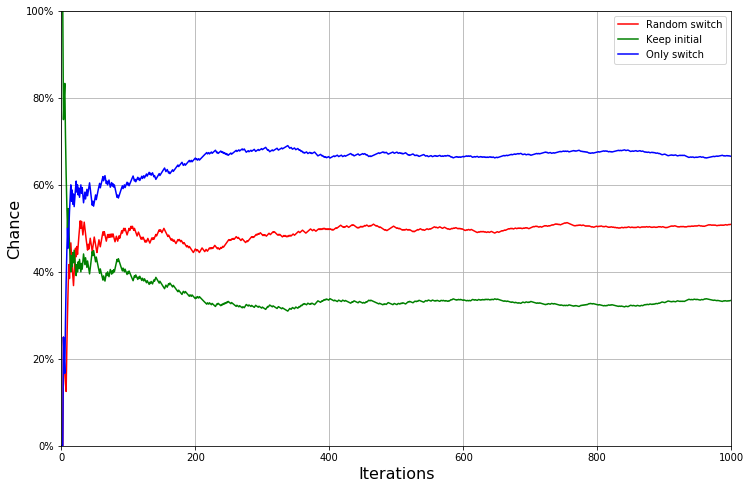# Monty Hall Paradox Simulation in Python¶

In :
from random import randint
import matplotlib.pyplot as plt
import matplotlib.ticker as ticker


This function will generate random rounds for our game. Each round consists of 3 doors. Only one of the doors is correct, other two are wrong.

In :
def generate_game(n: int):
game = []

for _ in range(n):
doors = [False] * 3
winner = randint(0, 2) # Choose winning door by random
doors[winner] = True
game.append(doors)

return game


This is a helper function that takes a list of 3 doors, looks at the second and third door, and then opens the one with goat (i.e. wrong door). This simulates a host with knowledge of what is behind the doors.

In :
def reveal_goat(doors):
# Get from doors 2 and 3 the one which contains goat.
for i in range(1, 3):
if doors[i] == False:
return i


Simulate random choice

Simulate situation where the player randomly chooses whether to keep his initial choice or switch his choice.

In :
def simulate_random_choice(game: list):
wins = 0
attempts = 0

history = []

for doors in game:
attempts += 1

# Host reveals a door with goat.
goat = reveal_goat(doors)

# Player randomly chooses whether to keep initial choice or switch.
new_choice = randint(0, 1)
final_choice = 0 if new_choice == 0 else 2 if goat == 1 else 1

if (doors[final_choice] == True):
wins += 1

history.append(wins / attempts)

return wins, history


Simulate initial choice

Simulate situation where the player only keeps his initial choice and never switches.

In :
def simulate_keep_choice(game: list):
wins = 0
attempts = 0
history = []

for doors in game:
attempts += 1

# User does not switch game.
if (doors == True):
wins += 1

history.append(wins / attempts)

return wins, history


Simulate switch choice

Simulate situation where the player switches his choice everytime.

In :
def simulate_switch_choice(game: list):
wins = 0
attempts = 0
history = []

for doors in game:
attempts += 1

# Host reveals a door with goat.
goat = reveal_goat(doors)

# Player switches his doors (here he chooses the non-opened doors).
new_choice = 1 if goat == 2 else 2

if (doors[new_choice] == True):
wins += 1

history.append(wins / attempts)

return wins, history


Now the computing begins. This generates $n$ random games for simulation.

In :
game = generate_game(1000)


Run the three simulations defined above for the generated game.

In :
wins_random, history_random = simulate_random_choice(game)
wins_keep, history_keep = simulate_keep_choice(game)
wins_switch, history_switch = simulate_switch_choice(game)


And finally, create some fancy graph so we can actually see the result.

In :
plt.figure(figsize=(12,8))
plt.plot(history_random, 'r', label="Random switch")
plt.plot(history_keep, 'g', label="Keep initial")
plt.plot(history_switch, 'b', label="Only switch")
plt.legend(loc='upper right')
plt.ylim(0, 1.0)
plt.xlim(0, 1000)
plt.ylabel("Chance", fontsize=16)
plt.gca().yaxis.set_major_formatter(ticker.PercentFormatter(xmax=1.0))
plt.xlabel("Iterations", fontsize=16)
plt.grid(True)
plt.show()